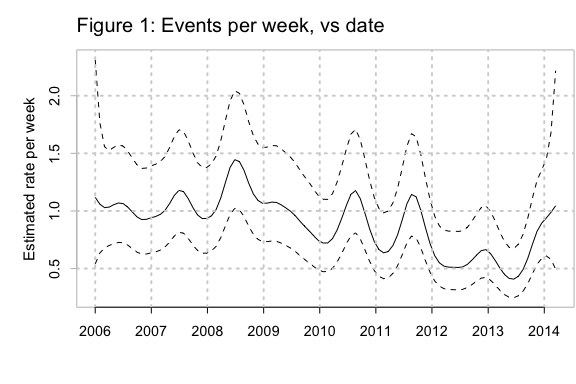# Prepare data – Weekly and daily counts

The interest is in broad patterns over time. Weekly counts give numbers that are easier to work with.

We use the function gamclass::eventCounts() to create counts of accidents, i) by week, and ii) by day, in both cases from January 1, 2006:

airAccs <- gamclass::airAccs
fromDate <- as.Date("2006-01-01")
dfDay06 <- gamclass::eventCounts(airAccs, dateCol="Date",
from= fromDate, by="1 day",
prefix="num")
dfDay06$day <- julian(dfDay06$Date, origin=fromDate)
dfWeek06 <- gamclass::eventCounts(airAccs, dateCol="Date", from=fromDate,
by="1 week", prefix="num")
dfWeek06$day <- julian(dfWeek06$Date, origin=fromDate)

# Fits, to the daily data, and to the weekly data

Figure 1 shows the fits to the weekly data:

cap1 <- "Figure 1: Fitted number of events (aircraft accidents) per week
versus time."
library(grid)
library(mgcv)
Loading required package: nlme
This is mgcv 1.8-42. For overview type 'help("mgcv-package")'.
year <- seq(from=fromDate, to=max(dfDay06\$Date), by="1 year")
atyear=julian(year, origin=fromDate)
dfWeek06.gam <- gam(num~s(day, k=200), data=dfWeek06, family=quasipoisson)
av <- mean(predict(dfWeek06.gam))
plot(dfWeek06.gam, xaxt="n", shift=av, trans=exp, rug=FALSE,
xlab="", ylab="Estimated rate per week", fg='gray')
axis(1, at=atyear, labels=format(year, "%Y"))
grid(lw=2,nx=NA,ny=NULL)
abline(v=atyear, lwd=2, lty=3, col='lightgray')
mtext(side=3, line=0.75, "Figure 1: Events per week, vs date", cex=1.25, adj=0)Figure 1: Fitted number of events (aircraft accidents) per week versus time.

The fitted curve and confidence band that results from modeling and plotting events per day is very similar. Code to plot events per day is:

cap2 <- "Figure 2: Fitted number of events (aircraft accidents) per day
versus time."
dfDay06.gam <- , out.width='60%', fig.width=6, fig.height=4, fig.cap=cap2}
gam(formula = num ~ s(day, k=200), family = quasipoisson,
data = dfDay06)
av <- mean(predict(dfDay06.gam))
plot(dfDay06.gam, xaxt="n", shift=av, trans=exp, rug=FALSE,
xlab="", ylab="Estimated rate per day", fg='gray')
axis(1, at=atyear, labels=format(year, "%Y"))
grid(lw=2,nx=NA,ny=NULL)
abline(v=atyear, lwd=2, lty=3, col='lightgray')
mtext(side=3, line=0.75, "A: Events per day, vs date", cex=1.25, adj=0)}